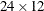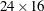### Example 59.1 Split-Plot Design

PROC MIXED can fit a variety of mixed models. One of the most common mixed models is the split-plot design. The split-plot design involves two experimental factors, A and B. Levels of A are randomly assigned to whole plots (main plots), and levels of B are randomly assigned to split plots (subplots) within each whole plot. The design provides more precise information about B than about A, and it often arises when A can be applied only to large experimental units. An example is where A represents irrigation levels for large plots of land and B represents different crop varieties planted in each large plot.

Consider the following data from Stroup (1989a), which arise from a balanced split-plot design with the whole plots arranged in a randomized complete-block design. The variable A is the whole-plot factor, and the variable B is the subplot factor. A traditional analysis of these data involves the construction of the whole-plot error (A*Block) to test A and the pooled residual error (B*Block and A*B*Block) to test B and A*B. To carry out this analysis with PROC GLM, you must use a TEST statement to obtain the correct F test for A.

Performing a mixed model analysis with PROC MIXED eliminates the need for the error term construction. PROC MIXED estimates variance components for Block, A*Block, and the residual, and it automatically incorporates the correct error terms into test statistics.

The following statements create a DATA set for a split-plot design with four blocks, three whole-plot levels, and two subplot levels:

data sp;
input Block A B Y @@;
datalines;
1 1 1  56  1 1 2  41
1 2 1  50  1 2 2  36
1 3 1  39  1 3 2  35
2 1 1  30  2 1 2  25
2 2 1  36  2 2 2  28
2 3 1  33  2 3 2  30
3 1 1  32  3 1 2  24
3 2 1  31  3 2 2  27
3 3 1  15  3 3 2  19
4 1 1  30  4 1 2  25
4 2 1  35  4 2 2  30
4 3 1  17  4 3 2  18
;


The following statements fit the split-plot model assuming random block effects:

proc mixed;
class A B Block;
model Y = A B A*B;
random Block A*Block;
run;


The variables A, B, and Block are listed as classification variables in the CLASS statement. The columns of model matrixconsist of indicator variables corresponding to the levels of the fixed effects A, B, and A*B listed on the right side of the MODEL statement. The dependent variable Y is listed on the left side of the MODEL statement.

The columns of the model matrixconsist of indicator variables corresponding to the levels of the random effects Block and A*Block. Thematrix is diagonal and contains the variance components of Block and A*Block. Thematrix is also diagonal and contains the residual variance.

The SAS statements produce Output 59.1.1Output 59.1.8.

The Model Information table in Output 59.1.1 lists basic information about the split-plot model. REML is used to estimate the variance components, and the residual variance is profiled from the optimization.

Output 59.1.1: Results for Split-Plot Analysis

The Mixed Procedure

Model Information
Data Set WORK.SP
Dependent Variable Y
Covariance Structure Variance Components
Estimation Method REML
Residual Variance Method Profile
Fixed Effects SE Method Model-Based
Degrees of Freedom Method Containment

The Class Level Information table in Output 59.1.2 lists the levels of all variables specified in the CLASS statement. You can check this table to make sure that the data are correct.

Output 59.1.2: Split-Plot Example (continued)

Class Level Information
Class Levels Values
A 3 1 2 3
B 2 1 2
Block 4 1 2 3 4

The Dimensions table in Output 59.1.3 lists the magnitudes of various vectors and matrices. Thematrix is seen to be, and thematrix is.

Output 59.1.3: Split-Plot Example (continued)

Dimensions
Covariance Parameters 3
Columns in X 12
Columns in Z 16
Subjects 1
Max Obs Per Subject 24

The Number of Observations table in Output 59.1.4 shows that all observations read from the data set are used in the analysis.

Output 59.1.4: Split-Plot Example (continued)

Number of Observations
Number of Observations Used 24
Number of Observations Not Used 0

PROC MIXED estimates the variance components for Block, A*Block, and the residual by REML. The REML estimates are the values that maximize the likelihood of a set of linearly independent error contrasts, and they provide a correction for the downward bias found in the usual maximum likelihood estimates. The objective function is –2 times the logarithm of the restricted likelihood, and PROC MIXED minimizes this objective function to obtain the estimates.

The minimization method is the Newton-Raphson algorithm, which uses the first and second derivatives of the objective function to iteratively find its minimum. The Iteration History table in Output 59.1.5 records the steps of that optimization process. For this example, only one iteration is required to obtain the estimates. The Evaluations column reveals that the restricted likelihood is evaluated once for each of the iterations. A criterion of 0 indicates that the Newton-Raphson algorithm has converged.

Output 59.1.5: Split-Plot Analysis (continued)

Iteration History
Iteration Evaluations -2 Res Log Like Criterion
0 1 139.81461222
1 1 119.76184570 0.00000000

 Convergence criteria met.

The REML estimates for the variance components of Block, A*Block, and the residual are 62.40, 15.38, and 9.36, respectively, as listed in the Estimate column of the Covariance Parameter Estimates table in Output 59.1.6.

Output 59.1.6: Split-Plot Analysis (continued)

Covariance Parameter Estimates
Cov Parm Estimate
Block 62.3958
A*Block 15.3819
Residual 9.3611

The Fit Statistics table in Output 59.1.7 lists several pieces of information about the fitted mixed model, including the residual log likelihood. The Akaike (AIC) and Bayesian (BIC) information criteria can be used to compare different models; the ones with smaller values are preferred. The AICC information criteria is a small-sample bias-adjusted form of the Akaike criterion (Hurvich and Tsai, 1989).

Output 59.1.7: Split-Plot Analysis (continued)

Fit Statistics
-2 Res Log Likelihood 119.8
AIC (smaller is better) 125.8
AICC (smaller is better) 127.5
BIC (smaller is better) 123.9

Finally, the fixed effects are tested by using Type 3 estimable functions (Output 59.1.8).

Output 59.1.8: Split-Plot Analysis (continued)

Type 3 Tests of Fixed Effects
Effect Num DF Den DF F Value Pr > F
A 2 6 4.07 0.0764
B 1 9 19.39 0.0017
A*B 2 9 4.02 0.0566

The tests match the one obtained from the following PROC GLM statements:

proc glm data=sp;
class A B Block;
model Y = A B A*B Block A*Block;
test h=A e=A*Block;
run;


You can continue this analysis by producing solutions for the fixed and random effects and then testing various linear combinations of them by using the CONTRAST and ESTIMATE statements. If you use the same CONTRAST and ESTIMATE statements with PROC GLM, the test statistics correspond to the fixed-effects-only model. The test statistics from PROC MIXED incorporate the random effects.

The various inference space contrasts given by Stroup (1989a) can be implemented via the ESTIMATE statement. Consider the following examples:

proc mixed data=sp;
class A B Block;
model Y = A B A*B;
random Block A*Block;
estimate 'a1 mean narrow'
intercept 1 A 1 B .5 .5 A*B .5 .5 |
Block     .25 .25 .25 .25
A*Block   .25 .25 .25 .25 0 0 0 0 0 0 0 0;

estimate 'a1 mean intermed'
intercept 1 A 1 B .5 .5 A*B .5 .5 |
Block     .25 .25 .25 .25;
intercept 1 a 1 b .5 .5 A*B .5 .5;
run;


These statements result in Output 59.1.9.

Output 59.1.9: Inference Space Results

The Mixed Procedure

Estimates
Label Estimate Standard Error DF t Value Pr > |t|
a1 mean narrow 32.8750 1.0817 9 30.39 <.0001
a1 mean intermed 32.8750 2.2396 9 14.68 <.0001
a1 mean broad 32.8750 4.5403 9 7.24 <.0001

Note that all the estimates are equal, but their standard errors increase with the size of the inference space. The narrow inference space consists of the observed levels of Block and A*Block, and the t-statistic value of 30.39 applies only to these levels. This is the same t statistic computed by PROC GLM, because it computes standard errors from the narrow inference space. The intermediate inference space consists of the observed levels of Block and the entire population of levels from which A*Block are sampled. The t-statistic value of 14.68 applies to this intermediate space. The broad inference space consists of arbitrary random levels of both Block and A*Block, and the t-statistic value of 7.24 is appropriate. Note that the larger the inference space, the weaker the conclusion. However, the broad inference space is usually the one of interest, and even in this space conclusive results are common. The highly significant p-value for ’a1 mean broad’ is an example. You can also obtain the ’a1 mean broad’ result by specifying A in an LSMEANS statement. For more discussion of the inference space concept, see McLean, Sanders, and Stroup (1991).

The following statements illustrate another feature of the RANDOM statement. Recall that the basic statements for a split-plot design with whole plots arranged in randomized blocks are as follows.

proc mixed;
class A B Block;
model Y = A B A*B;
random Block A*Block;
run;


An equivalent way of specifying this model is as follows:

proc mixed data=sp;
class A B Block;
model Y = A B A*B;
random intercept A / subject=Block;
run;


In general, if all of the effects in the RANDOM statement can be nested within one effect, you can specify that one effect by using the SUBJECT= option. The subject effect is, in a sense, factored out of the random effects. The specification that uses the SUBJECT= effect can result in faster execution times for large problems because PROC MIXED is able to perform the likelihood calculations separately for each subject.